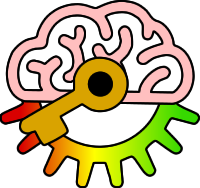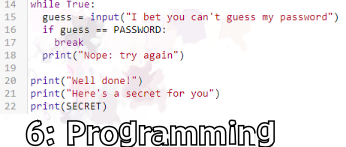# Edexcel GCSE Computer Science 2020

6.5.2: be able to write programs that use relational operators (equal to, less than, greater than, not equal to, less than or equal to, greater than or equal to)

Keywords:Test your self on these keywords and definitions using the games below or
KeywordDefinition
!=relational operator which evaluates to True if two values are not equal
<relational operator which evaluates to True if the first value is less than the second
<=relational operator which evaluates to True if the first value is less than or equal to the second
==relational operator which which evaluates to True if two values are equal
>relational operator which evaluates to True if the first value is less than the second
>=relational operator which evaluates to True if the first value is greater than or equal to the second
relational operatorsymbol or characters which compare two values giving the result as a boolean True or False
Keyword games:
Resources: